# Multiplying Fractions by Whole Numbers 9-9

##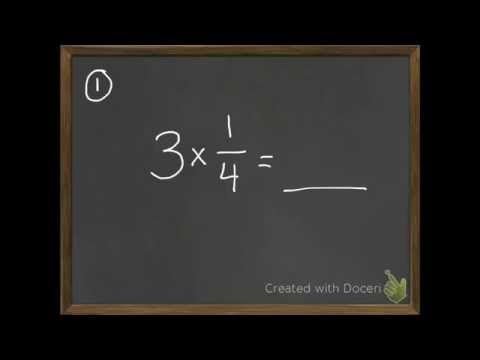By David Horan

multiply a fraction with a whole number# Multiplying Fractions by Whole Numbers 9-9

##By David Horan

multiply a fraction with a whole number.# Multiplying by 10

##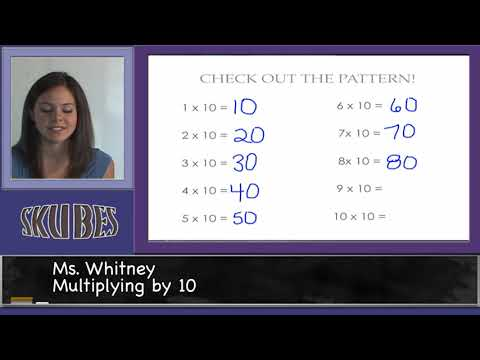By Skubes ed

Multiply one-digit whole numbers by multiples of 10 in the range 10–90 (e.g., 9 × 80, 5 × 60) using strategies based on place value and properties of operations.# Properties and patterns for multiplication | 3rd grade | Khan Academy

##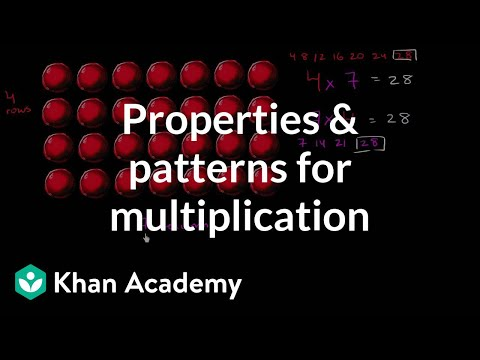By Khan Academy

Change the order of numbers or decompose numbers to simplify multiplication problems.# Fundamental Theorem of Algebra for quadratic | Algebra II | Khan Academy

##By AbsoluteMathematics

This video offers a step-by-step sample problem of Polynomials and the Fundamental Theorem of Algebra.# [3.NBT.3-1.0] Intro multiplication - Common Core Standard

##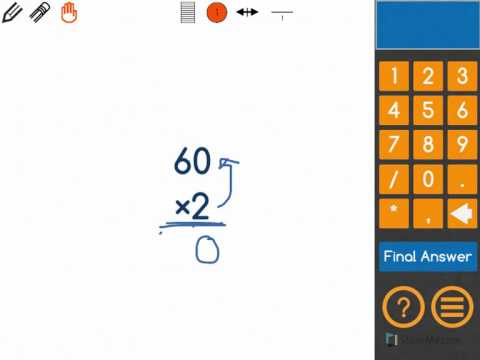By Freckle by Renaissance

Multiply one-digit whole numbers by multiples of 10 in the range 10–90 (e.g., 9 × 80, 5 × 60) using strategies based on place value and properties of operations. Front Row is a free, adaptive, Common Core aligned math program for teachers and students in kindergarten through eighth grade. Front Row allows students to practice math at their own pace - learning advanced concepts when they're ready and receiving remediation when they struggle. Front Row provides teachers with access to a detailed data dashboard and weekly email reports that show which standards are causing students difficulty, what small groups can be formed for interventions, and how their students are progressing in math.# Patterns in multiplication tables

##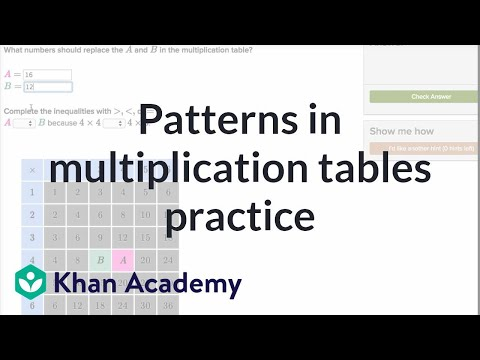By Khan Academy

Read a multiplication table, and fill in the missing pieces.# Complex Numbers In Polar Form De Moivre's Theorem, Products, Quotients, Powers, and nth Roots Prec

##By The Organic Chemistry Tutor

This precalculus video tutorial focuses on complex numbers in polar form and de moivre's theorem. It explains how to find the products, quotients, powers and nth roots of complex numbers in polar form as well as converting it to and from rectangular form. This video contains plenty of examples and practice problems and is useful for high school and college students taking precalculus or trigonometry. Here is a list of topics: 1. Graphing / Plotting Complex Numbers in a Complex Plane 2. Real Axis vs Imaginary Axis 3. How To Find The Absolute Value of a Complex Number 4. Complex Numbers - Rectangular Form to Polar Form 5. Converting Complex Numbers in Polar Form To Rectangular Form 6. Complex Numbers - List of Equations and Formulas 7. Finding R and Angle Theta From a and b 8. Writing Complex Numbers In Rectangular Form 9. Product of Two Complex Numbers In Polar Form Equation 10. Quotient of Two Complex Numbers In Polar Form Formula 11. Finding Products of Complex Numbers in Polar Form 12. Finding Quotients of Complex Numbers in Polar Form 13. Powers of Complex Numbers in Polar Form 14. De Moivre's Theorem - Roots of Complex Numbers in Polar Form 15. Solving Equations With Complex Numbers 16. Adding Complex Numbers in Polar Form 17. Multiplying Complex Numbers in Polar Form 18. Dividing Complex Numbers in Polar Form# 3rd Grade Math Rap

##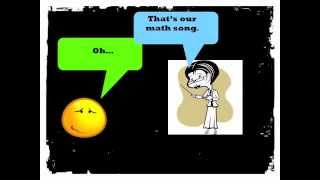By McCarthy Math Academy

With a little help from the group, Mindless Behavior, I have created a math video with lyrics to help my students to understand and apply core math skills for third grade. People of all ages can jam out to this one. Enjoy!

I've got a case of the operation blues.
Because I don't know which one I should use.
Look at the word problem for the clues.
The key words tell you how to choose.

Each means you multiply or you must divide.
Tryna find the total? Then you multiply
Total's in the problem? Then you must divide.
Not quick to solve it, draw it, get it right.

Addition's easy for me and you
Sum, In all, together, and total too.
When do you subtract? How many more?
Fewer? Left? Less? Difference in a score?

Place value's next. Disco on the " dess "
Ones, tens, hundreds, to the left
Thousands, Ten thousands, hundred...thousand
Say the name of the place, yeah.

The value's the amount of the place
For example, 2,060.
The value of the 2 is 2-0-0-0,
The value of the 6 is 6-0.

When you round, find and underline the place
Spotlight to the right, decide the digit's fate
5 or more, add 1 to the rounding place
4 or less, do nothing but walk away, (estimate)

A pen, penny is one, one cent
A Nic-kel is five, a dime is ten cents
25 for a quarter, George Washington
100 cents makes a dollar, there he goes again.

For pictographs, you gotta check out the key
One smiley face might really equal three
For bar graphs, pay attention to the scale
Think it's counting by ones, huh, you'll fail

Fractions are easy, just draw your best.
Here they go from least to greatest
1/12, 1/6, Â¼, 1/3,
Â½, 2/3, Â¾, Fraction nerd!

You see that number on top,
That's called the numerator
It describes the amount
That is being considered
And if you jump down from the fraction bar
Denominator
It's the total number of equal parts.

Let me give you an example:
Leslie Moin has some coins
A total of 9
2 happen to be pennies
While 7 are dimes.
What's the fraction of dimes?
How many coins? 9
How many dimes? 7
Say the fraction -- seven ninths

Length times width is Area
Distance around is Perimeter
Break down the GEOMETRY

3 sides makes triangle
4 sides = quadrilateral
5 pentagon, 6 hexagon
8 octagon, 10 decagon

Lines that never cross - PARALLEL
Lines that meet or cross - INTERSECTING
Lines that form right angles -- PERPENDICULAR

Same shape, same size -- CONGRUENT
Line that cuts in half - SYMMETRY
Up and Down - VERTICAL
Left to Right -- HORIZONTAL

An angle less than right - ACUTE
An angle opened wide - OBTUSE
Ninety degrees square corner - RIGHT ANGLE

Back to triangles
3 sides the same = equilateral
2 sides the same = isosceles
no sides the same = Hey, that's a scalene right!

So, that's it.
That's our math song.
Before we leave,
Remember to read
Your math problems three times before you answer.
That way you know what the problem
Is asking you to do.
Don't be lazy, be brilliant.
Piece! Like a fraction.# ALL OF GRADE 9 MATH IN 60 MINUTES!!! (exam review part 1)

##By Lumos Learning

Here is a great exam review video reviewing all of the main concepts you would have learned in the MPM1D grade 9 academic math course. The video is divided in to 3 parts. This is part 1: Algebra. The main topics in this section are exponent laws, polynomials, distributive property, and solving first degree equations. Please watch part 2 and 3 for a review of linear relations and geometry. If you watch all 3 parts, you will have reviewed all of grade 9 math in 60 minutes. Enjoy! Visit jensenmath.ca for more videos and course materials.# 1.8 - Finding the inverse of a rational function with a root as a denominator - coolmath

##By Mr. McLogan's Math Channel

In this video tutorial I will show you how to find the inverse of a function f(x). I do this by first writing the equation substituting y for f(x). I then solve the equation for x. The result is the inverse. The inverse can be written, substituting y back in for x, and x for y. I also show how to verify that a function is an inverse of another function. I am a math teacher that provides free online math tutoring by working through problems for the website www.freemathvideos.com These questions are usually to help students understand their math homework or to do well on their math test. The videos are made for students that want help with math and want to be shown a step by step process into solving the problems. In addition to solving the problems I also offer explanations into why I am doing each step. I offer free math videos so that my students can learn math online if they missed a day of school. However I also like to answer questions that are no# Math Antics - Adding Mixed Numbers

##By# 4th Grade Math

##By Lumos Learning

Grade 4 lesson demonstrating CCSS Math Standard 4.OA..A.1,2, using literacy strategies to understand word problems. Lesson progresses through prior understandings of multiplication as groups of objects to multiplicative comparisons.# 4th Grade Math

##By

Grade 4 lesson demonstrating CCSS Math Standard 4.OA..A.1,2, using literacy strategies to understand word problems. Lesson progresses through prior understandings of multiplication as groups of objects to multiplicative comparisons.# Financing California: K-12 Education

##By Lumos Learning

Spending on K-12 education accounts for 40% of California's budget. With spending per capita and performance falling, a panel of experts discusses the future of K-12 education. Series: "Travers Conference in Ethics and Accountability# 2011 Roundtable at Stanford: Redefining K-12 Education in America

##By Lumos Learning

October 22, 2011 - Designing an education that truly builds the necessary skills for today's enormously diverse student population is not easy. But it's the key to opportunity for our citizens, economic vitality for our nation, and to assuring the U.S. remains a world leader. There is hope: innovations and innovators that challenge the status quo; research to help us understand how to move the education needle; a virtual army of reformers experimenting with new ways to teach, learn, and run our public schools.